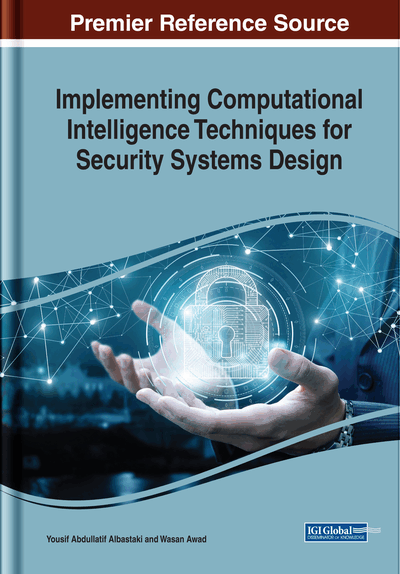# Composite Discrete Logarithm Problem and a Reconstituted ElGamal Cryptosystem Based on the Problem: New ElGamal Cryptosystems With Some Special Sequences and Composite ElGamal Cryptosystem

Çağla Özyılmaz (Ondokuz Mayıs University, Turkey) and Ayşe Nallı (Karabuk University, Turkey)
DOI: 10.4018/978-1-7998-2418-3.ch008

## Abstract

In this chapter, the authors have defined a new ElGamal cryptosystem by using the power Fibonacci sequence module m. Then they have defined a new sequence module m and the other ElGamal cryptosystem by using the new sequence. In addition, they have compared that the new ElGamal cryptosystems and ElGamal cryptosystem in terms of cryptography. Then the authors have defined the third ElGamal cryptosystem. They have, particularly, called the new system as composite ElGamal cryptosystem. The authors made an application of composite ElGamal cryptosystem. Finally, the authors have compared that composite ElGamal cryptosystem and ElGamal cryptosystem in terms of cryptography and they have obtained that composite ElGamal cryptosystem is more advantageous than ElGamal cryptosystem.
Chapter Preview
Top

## Introduction

The fundamental objective of cryptography is to enable two people, which are usually referred to as Alice and Bob, to communicate over an insecure channel in such a way that an opponent, Oscar, can’t understand what is being said. The information that Alice wants to send to Bob, which is called ‘plaintext’, can be English text, numerical data, or anything else, that is, its structure is selected arbitrarily. Alice encrypts the plaintext, using a predetermined key, and sends ciphertext over the insecure channel. Oscar, by seeing the ciphertext in the channel, can’t determine what the the message was; but Bob who knows the encryption key, can decrypt the ciphertext and revive the plaintext or the message. These situations are identified by using the following mathematical notation.

Definition 1. A cryptosystem is a five–tuplewhere the following conditions are satisfied:

In practice, there are two types of cryptosystems.

• 1.

Symmetric Cryptography (Secret key cryptosystems)

• 2.

Asymmetric Cryptography (Public key cryptosystems)

Normally, in any cryptosystem, the encryption and the decryption key are closely related. It is practically impossible to decrypt the ciphertext with the key that is unrelated to the encryption key. In symmetric cryptography is used a single key for both encryption and decryption and in asymmetric cryptography, such as the RSA (Rivest et al., 1978) and ElGamal cryptosystem (ElGamal, 1985), is used different keys for encryption and decryption (Hwang et al., 2002).

In this chapter, the authors have focused on asymmetric cryptography and they, especially, have cited that discrete logarithm problem which is one of the mathematical difficult problems that are used in asymmetric cryptography and ElGamal cryptosystem based on the discrete logarithm problem. They have defined new discrete logarithm problems and new ElGamal cryptosystem and they have made new applications.

Top

## Background

At the very heart of cryptography is the notion of one way function, which was shown to be necessary and sufficient for many cryptographic primitives.

## Key Terms in this Chapter

Fibonacci Sequence: It is one of the most famous formulas such that every number in the sequence is the sum of the two consecutive numbers.

Public Key Cryptosystem: It is an encryption system that uses two keys such that one of them is public key and the other is private key and it is computationally infeasible compute the private key.

Composite Number: It is a positive integer that has at least one divisor other than 1 and itself.

One Way Function: It is a function that is easy to compute on every input, but it is hard to invert given the image of a random input.

Trapdoor: It is a special information which is used to find inverse.

Cryptography: It is a process of protecting information and communications such that the only one for whom the information is intended can understand.

## Complete Chapter List

Search this Book:
Reset